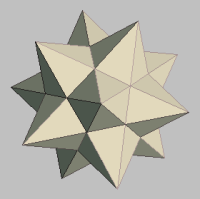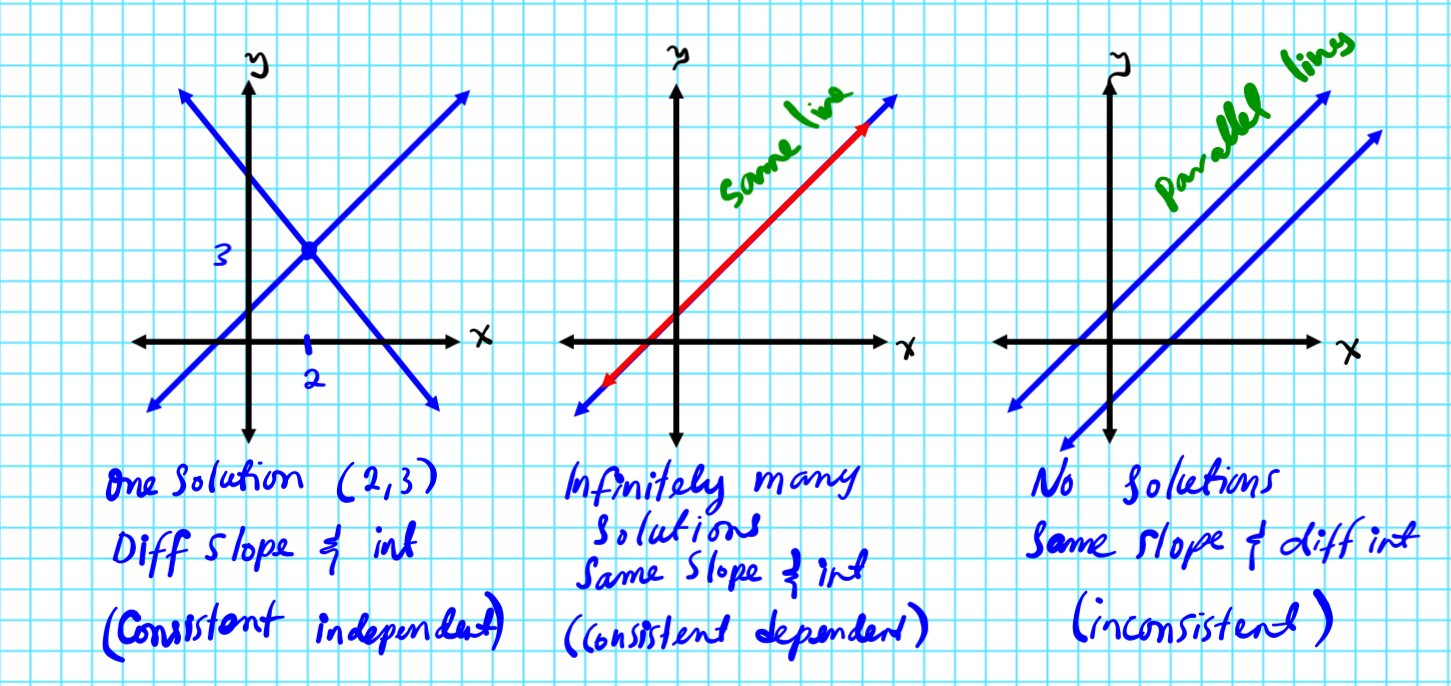MATHGOTSERVEDSolving System of Equations
Learning Objective
On this page you will learn how to solve systems of two equations in two variables by:
1) Graphing
2) Linear Combination or Elimination
3) Substitution
Definition
A system of equation in two variables consists of two linear equations of the form

ax+by=c
dx+ey=f

The solution is the ordered pair (x,y) that satisfies both equations.

Example:
2x-y=6
x+3y=10

The solution is (4,2) since the ordered pair makes both equations true.

Solving Systems of two Linear Equations  by Graphing
How to Solve of Systems of Two Linear Equations by Graphing (Steps)
1. Graph the two equations using intercepts (standard form: Ax+By=C), or the slope m, and intercept b (slope intercept form y=mx+b).
2. Find the coordinates of the point of intersection of the two lines.
3. You should have one of the possible solutions
a) The two lines intersect at one point (different slope & intercept) => One             solution
b) The two lines lie on top of one another (same slope & intercept) =>                      Infinitely many solutions
c) The two lines are parallel and never intersect (same slope & different                     intercept) => No solutions

mathgotserved.comSolving System of Equations by Elimination (Linear Combination)
When to Use the Method of Elimination:
The method of solving linear systems by elimination is best suited for systems provided in standard form (Ax+By=C), or a situation where it is easy to place both equations in standard form (Ax+By=C).
How to Solve Systems Using the Method of Elimination (Steps):

1. Place both equations in standard form (Ax+By=C)  if they are not already in that form.
2. Identify the variable terms that are opposite of one another or the easiest to make into opposites. (Hint if like variable terms are different use the LCD  of the terms. )
3. Eliminate the like variables that are opposites by adding the two equations.
4. Isolate the remaining variable for the first solution.
5. Substitute the solution into one of the two original equations to solve for the eliminated variable.
​                                                                                         mathgotserved.com
Practice Test
Solution Practice Test